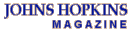Johns Hopkins Magazine -- April 1997APRIL 1997CONTENTS RETURN TO TEST YOUR SITING SAAVY AUTHOR'S NOTEBOOK
Query #2

If you studied math in college and are familiar with the concept of the "centroid," you probably answered b. (On a line with weighted populations, the centroid is the position at which an imaginary bar would balance horizontally.)

But that answer is based on a misconception that's been around for 70 years--a misconception that came out of a Bureau of the Census report in the 1920s. The report suggested that the point that minimized the sum of people's travel to the central facility was the centroid. In fact, it isn't.

The better answer is a) within one of the towns. (There could be locations at more than one town that give the same minimum, and there could be an additional location not at a town, but always there will be an answer within at least one of the towns.) Allow me to illustrate:

Let's say towns A, B, and C are at points on a single line, with student populations of 160, 200, and 100, respectively. Town B is 20 miles from Town A, and Town C is 10 miles from Town B.

First, let's find the centroid, or balance point for the line. We will say it is Y distance to the right of A. The centroid is shown by the fulcrum symbol. To make the system balance, we multiply the distance from one town to the next by the number of students who will travel that distance:

160Y = 200(20-y) + 100[10+(20-Y)]

160Y = 4,000 -200Y +100 [30-Y]

160Y = 4,000 + 3,000 -200Y -100Y

460Y = 7,000

Y = 15.22 miles

So, if the fulcrum is 15.22 miles to the right of A, what is the total pupil miles to a school located at the fulcrum or centroid? It is 160 x 15.22 (the travel by students at A) + 200 x (20-15.22) (the travel by students at B) + 100 x (10 + (20-15.22)) or 4,869 student miles.

My claim is that even for this little example, siting the school at one of the nodes, or towns, will reduce aggregate travel to less than what it would be if the centroid were used as the central spot for the grade school.

If the school is at town A, total travel =
200 x 20 + 100 x 30= 7,000 student miles

If the school is at town B, total travel =
160 x 20 +100 x 10= 4,200 student miles

If the school is at town C, total travel =
160 x 30 + 200 x 10= 6,800 student miles

As you can see, total student miles is least at point B (4,200), and less than the travel would be if the school were at the centroid or at either town A or C. In fact, it is the lowest it can be. No matter where on the line you try to locate the grade school, you can't get lower aggregate travel. Try it. In fact, try any network of nodes and lines joining the nodes.

Let me be more specific. Using a ruler, create any network of nodes and the lines joining the nodes. Don't let the lines cross, or you'll create a new node with which to contend. Use whole number distances for the length of the lines, and make your drawing in proportion to those whole number distances (i.e., convert 40 miles to 4 inches, etc.) Don't forget to implement the principle of the triangle inequality (the sum of the lengths of any two sides of a triangle is greater than the length of the third side). That's a property of real-life networks, so we want it to hold for sure in terms of the distance labels you put on your drawing. Attach populations to each node (small whole numbers make for easier calculations). If you don't want to do this you can use my drawing below:

Now, calculate the total population times distance to each of the four nodes. If A is the facility, the population at A doesn't travel, so its population times distance is zero. Find the node which, when it is designated as the facility, gives the lowest total student travel.

Now for the challenge. Try another point on the network that is not on a node. If you can find a point on the network that gives a lower value of total travel than that of a node, I will... I will... be very surprised.